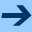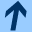Example

## Example

### Original AUTOC file

Here is an example of an AUTOC automaton specification, corresponding to the scheduler of .

```
-- channels are first declared
channel I1();
channel I2();
channel start(); -- used to simulate an initial transition

process I { -- Models a non-deterministic environment
sync I1,I2,start; -- set of channels on which the process synchronizes
var c1,c2 : real; -- set of variables of the process
init pc=linit;    -- initial condition, using the implictly declared
-- pc variable, of enumerated type
loc linit:
continuous blocked; -- time is not allowed to elapse here
when c1>=0 and c2>=0 sync start() goto l;
-- guarded transition with synchronization
loc l:
continuous c1=1 and c2=1; -- defines the derivatives of c1 and c2 to be equal to 1
when c1>=10 sync I1() goto l assign { c1 := 0 };
when c2>=20 sync I2() goto l assign { c2 := 0 };
}

process T { -- The scheduler itself
sync E1, E2, I1, I2, start;
var x1,x2 : real;
k1,k2 : int;
init pc=linit;
loc linit:
continuous blocked;
when x1=0 and x2=0 and k1=0 and k2=0 sync start() goto Idle;
loc Idle:
continuous x1=0 and x2=0;
when true sync I1() goto Task1 assign { k1:=1 };
when true sync I2() goto Task2 assign { k2:=1 };
continuous if x1<=4 then x1=1 and x2=0 else blocked;
-- The guard "x1<=4" is here the ``invariant'' of the location.
-- If it is not satisfied, the time is blocked.
when x1<4 sync I1() goto Task1 assign { k1 := k1 + 1 };
when x1<4 sync I2() goto Task2 assign { k2 := 1 };
when x1=4 and k1<=1 goto Idle assign { k1 := k1-1; x1 := 0 };
when x1=4 and k1>=2 goto Task1 assign { k1 := k1-1; x1 := 0 };
continuous if x2<=8 then x1=0 and x2=1 else blocked;
when x2<8 sync I1() goto Task2 assign { k1 := k1 + 1 };
when x2<8 sync I2() goto Task2 assign { k2 := k2 + 1 };
when x2=8 and k2<=1 and k1=0 goto Idle assign { k2 := k2-1; x2 := 0 };
when x2=8 and k2<=1 and k1>=1 goto Task1 assign { k2 := k2-1; x2 := 0 };
when x2=8 and k2>=2 goto Task2 assign { k2 := k2-1; x2 := 0 };
}

system I,T; -- Specifies the set of processes in the system
explicit T; -- Specifies the processes to be composed explicitly
#pragma final "not (I.pc=I.linit) and T.k2>=2" -- pragma clause, to be transmitted unmodified
```

### AUTO file generated by AUTOC2AUTO

Assuming the previous file is named scheduler.autc, the command
autoc2auto scheduler.autc scheduler.aut
or simply
autoc2auto scheduler.autc
produces the following file scheduler.aut:

```
type I.pc_t = enum { I.linit, I.l };

channel I1();
channel I2();
channel start();
channel T.tau(); -- for internal transitions of T

system (I,T) (T) {
-- The pair "(I,T)" allows to interpret transition degrees (see below)
-- "(T)" means that only T is explicit in the product, and allows to interpret location names

sync I1, I2, start;
var I.c1 : real;
I.c2 : real;
I.pc : I.pc_t;
T.pc : T.pc_t;
T.x1 : real;
T.x2 : real;
T.k1 : int;
T.k2 : int;

init I.pc = I.linit and T.pc = T.linit;
loc (linit):
continuous blocked;
(0,0): -- Transition degree: this transition is the composition of
-- the first transition of I starting from linit and synchronized on start
-- with the first transition of T starting from linit synchronized on start
when I.pc = I.linit and I.c1 >= 0 and I.c2 >= 0 and
T.x1 = 0 and T.x2 = 0 and T.k1 = 0 and T.k2 = 0
sync start()
goto (Idle)
assign { I.pc := if I.pc = I.linit and I.c1 >= 0 and I.c2 >= 0
then I.l
else I.pc };

loc (Idle):
continuous (if (I.pc = I.linit)
then blocked
else if (I.pc = I.l)
then I.c1 = 1 and I.c2 = 1
else blocked) and T.x1 = 0 and T.x2 = 0;
(0,0):
when I.pc = I.l and I.c1 - 10 >= 0
sync I1()
assign { I.c1 := if I.pc = I.l and I.c1 - 10 >= 0 then 0 else I.c1;
I.pc := if I.pc = I.l and I.c1 - 10 >= 0 then I.l else I.pc;
T.k1 := 1 };
(0,0):
when I.pc = I.l and I.c2 - 20 >= 0
sync I2()
assign { I.c2 := if I.pc = I.l and I.c2 - 20 >= 0 then 0 else I.c2;
I.pc := if I.pc = I.l and I.c2 - 20 >= 0 then I.l else I.pc;
T.k2 := 1 };

continuous (if (I.pc = I.linit)
then blocked
else if (I.pc = I.l)
then I.c1 = 1 and I.c2 = 1
else blocked) and
(if (4 - T.x1 >= 0) then T.x1 = 1 and T.x2 = 0 else blocked);
(0,0):
when I.pc = I.l and I.c1 - 10 >= 0 and 4 - T.x1 > 0
sync I1()
assign { I.c1 := if I.pc = I.l and I.c1 - 10 >= 0 then 0 else I.c1;
I.pc := if I.pc = I.l and I.c1 - 10 >= 0 then I.l else I.pc;
T.k1 := T.k1 + 1 };
(0,0):
when I.pc = I.l and I.c2 - 20 >= 0 and 4 - T.x1 > 0
sync I2()
assign { I.c2 := if I.pc = I.l and I.c2 - 20 >= 0 then 0 else I.c2;
I.pc := if I.pc = I.l and I.c2 - 20 >= 0 then I.l else I.pc;
T.k2 := 1 };
(0,0):
when T.x1 = 4 and 1 - T.k1 >= 0
sync T.tau() goto (Idle) assign { T.x1 := 0; T.k1 := T.k1 - 1 };
(0,1):
when T.x1 = 4 and T.k1 - 2 >= 0
sync T?tau() goto (Task1) assign { T.x1 := 0; T.k1 := T.k1 - 1 };

continuous (if (I.pc = I.linit)
then blocked
else if (I.pc = I.l)
then I.c1 = 1 and I.c2 = 1
else blocked) and
(if (8 - T.x2 >= 0) then T.x1 = 0 and T.x2 = 1 else blocked);
(0,0):
when I.pc = I.l and I.c1 - 10 >= 0 and 8 - T.x2 > 0
sync I1()
assign { I.c1 := if I.pc = I.l and I.c1 - 10 >= 0 then 0 else I.c1;
I.pc := if I.pc = I.l and I.c1 - 10 >= 0 then I.l else I.pc;
T.k1 := T.k1 + 1 };
(0,0):
when I.pc = I.l and I.c2 - 20 >= 0 and 8 - T.x2 > 0
sync I2()
assign { I.c2 := if I.pc = I.l and I.c2 - 20 >= 0 then 0 else I.c2;
I.pc := if I.pc = I.l and I.c2 - 20 >= 0 then I.l else I.pc;
T.k2 := T.k2 + 1 };
(0,0):
when T.x2 = 8 and 1 - T.k2 >= 0 and T.k1 = 0
sync T.tau() goto (Idle) assign { T.x2 := 0; T.k2 := T.k2 - 1 };
(0,1):
when T.x2 = 8 and 1 - T.k2 >= 0 and T.k1 - 1 >= 0
sync T.tau() goto (Task1) assign { T.x2 := 0; T.k2 := T.k2 - 1 };
(0,2):
when T.x2 = 8 and T.k2 - 2 >= 0
sync T.tau() goto (Task2) assign { T.x2 := 0; T.k2 := T.k2 - 1 };

}

#pragma final "not (I.pc=I.linit) and T.k2>=2" -- pragma clause, to be transmitted unmodified
```

A few explanations about how AUTOC2AUTO works:

• it introduces enumerated types for program counter variables, with the naming scheme P.pc_t.
• it possibly makes explicit the internal channel of each process (if there are internal transitions), with the naming scheme P.tau;
• it introduces new names for communication parameters;
• it labels the transitions of each process with a "degree", which is just an integer, the purpose of which is to resolve explicit non-determinism of transitions. For each location and each synchronization channel, the degree allows to distinguish the different transitions starting from this location and synchronized on the channel. This notion of degree can be useful for automatic tools, and is needed for the translation to NBAC which accepts only deterministic systems (with inputs).
• The product is then performed: locations of the result are the product of the explicitly composed process (explicit declaration in AUTOC format). The state and behaviour of other processes are encoded with their "pc" variables.

When one specifies a system directly with the AUTO format, degrees may be omitted.

### NBAC file generated by AUTO2NBAC

Assuming the previous file is named scheduler.aut, the command
auto2nbac scheduler.aut scheduler.ba
or simply
auto2nbac scheduler.aut
produces the file scheduler.ba. We display only parts of it:

```
typedef
I.pc_t = enum { I.linit, I.l };
channel_t = enum { I1, I2, start, T.tau }; -- type of the "channel" variable
state
I.c1 : real;
I.c2 : real;
I.pc : I.pc_t;
T.pc : T.pc_t;
T.x1 : real;
T.x2 : real;
T.k1 : int;
T.k2 : int;
input
channel : channel_t; -- used to encode synchronization channel
aux0 : uint;      -- used to encode the degree of process I
aux1 : uint;      -- used to encode the degree of process T
...
initial I.pc = I.linit and T.pc = T.linit;
space true;
final not I.pc = pc.linit and T.k1 - 3 >= 0; -- Content of the "final" pragma in Auto file

automaton -- initial control structure: the one defined by the Auto system
T.pc = T.Task2; -- definition of the location (a state formula)
location Idle_ :
T.pc = T.Idle;
location linit_ :
T.pc = T.linit;

channel = T.tau and
T.x2 = 8 and T.k2 - 2 >= 0 and aux0 = uint(0) and aux1 = uint(2) or
channel = I2 and
I.pc = I.l and I.c2 - 20 >= 0 and 8 - T.x2 > 0 and
aux0 = uint(0) and aux1 = uint(0) or
channel = I1 and
I.pc = I.l and I.c1 - 10 >= 0 and 8 - T.x2 > 0 and
aux0 = uint(0) and aux1 = uint(0)
-- first condition: necessary condition for a discrete transition to be fired
,
true
-- second condition: necessary condition for a continuous transition to be fired
;
...
```

### Annotating original AUTO file with the result delivered by NBAC

Let us perform a reachability analysis, trying to prove the property (that the final condition is not reachable) and outputting the set of reachable state to scheduler.aut.ba:
nbacg.opt -analysis 0 +success +valid -o scheduler.aut.ba scheduler.ba
and then annotate the result on the initial AUTO automaton by generating scheduler.aut.aut as follows:
nbac2auto -bdd scheduler.aut scheduler.aut.ba scheduler.aut.aut
We get this, that we may display with DOT by typing
auto2dot scheduler.aut.aut scheduler.dot
dot -Tps scheduler.dot >scheduler.ps
and we obtain this file.

Bertrand Jeannet, February 10, 2011Example